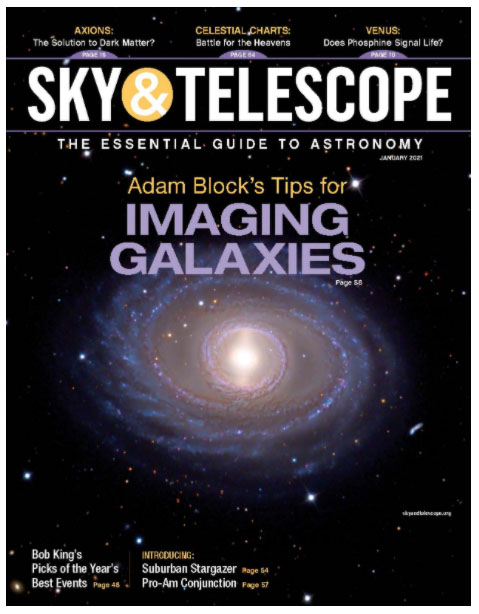January 2021 Issue!!The Normalize Scale Gradient script, by John Murphy,  represents a big step forward in producing the very best results in PixInsight. By normalizing images in a robust and consistent way improvements in pixel rejection, gradient simplification and most importantly proper image weighting are all fully realized. The following sections explain how to take advantage of this script and why it works.

 Introduction 13 minutes Finding Reference Frames 15 minutes First Look: Setup 15 minutes First Results! 17 minutes Second Important Example 12 minutes The Details Part 1 19 minutes The Details Part 2 30 minutes Two Data Sets Demo! 7 minutes

ERRATA:

After watching the videos the explanation of the errors and incomplete statements I made will make more sense.

1. In the video I say repeatedly that the weights are the inverse of the calculated scaling factor. This is an incomplete statement. Technically the weights are calculated as the combination of both measures of scaling factor AND noise calculations (MRS). The inverse of this term squared results in the weights. You can see this term in the explanations I give in the ImageIntegration section. It is this term that is problematic in the regular Noise Evaluation method- since the scaling factor is on the bottom in a squared term- any small error in this value results in a large error in the weight. Unfortunately the calculation of the scale in ImageIntegration is the issue- which is why this NSG script is so amazing.

2. At one point, I incorrectly say the gradient is calculated before the scaling factor. This is incorrect (and I say it correctly later). The scaling factor through the photometry of stars is calculated first. You need this to get the correct scaling value for the samples that are used to calculate the gradient. The order of operations is just as the name of the script implies.

3. I mention a detail that talks about adjusting the zone of avoidance around stars. I say the slider is a non-linear and the circle grows more quickly around bright stars. This is incorrect. The growth is linear with respect to the measured flux of the stars.

4. In the section I talk about the curvature files, I am wrong about a guess I made. I state that the curvature file is the residual of the averaged gradients of the target frames. This is incorrect. This is what happens when you guess! lol. The curvature image is what is left after subtracting the first "linear" term of the gradient. What we see left over is the "residual" from this tilted plane (linear gradient) across the field...and most importantly it is calculated (as is the case for all calculations) between the given target frame and the reference frame.

Thanks to John Murphy for correcting my errors. There are some others, but none too consequential. The above may not be perfect, but it is closer to the right way to describe the way the script works.